Next: Ampère's Circuital Law Up: Magnetism Previous: Charged Particle in a

## The Hall Effect

We have repeatedly stated that the mobile charges in conventional conducting materials are negatively charged (they are, in fact, electrons). Is there any direct experimental evidence that this is true? Actually, there is. We can use a phenomenon called the Hall effect to determine whether the mobile charges in a given conductor are positively or negatively charged. Let us investigate this effect.

Consider a thin, flat, uniform, ribbon of some conducting material which is orientated such that its flat side is perpendicular to a uniform magnetic field--see Fig. 26. Suppose that we pass a currentalong the length of the ribbon. There are two alternatives. Either the current is carried by positive charges moving from left to right (in the figure), or it is carried by negative charges moving in the opposite direction.

Suppose that the current is carried by positive charges moving from left to right. These charges are deflected upward (in the figure) by the magnetic field. Thus, the upper edge of the ribbon becomes positively charged, whilst the lower edge becomes negatively charged. Consequently, there is a positive potential difference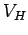between the upper and lower edges of the ribbon. This potential difference is called the Hall voltage.

Suppose, now, that the current is carried by negative charges moving from right to left. These charges are also deflected upward by the magnetic field. Thus, the upper edge of the ribbon becomes negatively charged, whilst the lower edge becomes positively charged. It follows that the Hall voltage (i.e., the potential difference between the upper and lower edges of the ribbon) is negative in this case.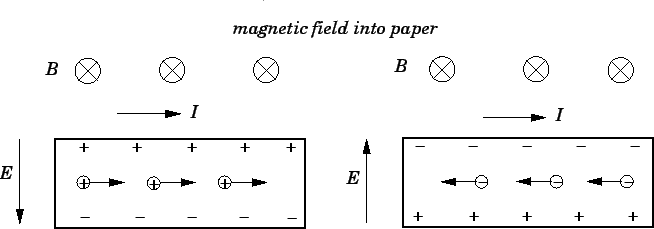Clearly, it is possible to determine the sign of the mobile charges in a current carrying conductor by measuring the Hall voltage. If the voltage is positive then the mobile charges are positive (assuming that the magnetic field and the current are orientated as shown in the figure), whereas if the voltage is negative then the mobile charges are negative. If we were to perform this experiment we would discover that the the mobile charges in metals are always negative (because they are electrons). However, in some types of semiconductor the mobile charges turn out to be positive. These positive charge carriers are called holes. Holes are actually missing electrons in the atomic lattice of the semiconductor, but they act essentially like positive charges.

Let us investigate the magnitude of the Hall voltage. Suppose that the mobile charges each possess a chargeand move along the ribbon with the drift velocity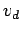. The magnetic force on a given mobile charge is of magnitude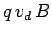, since the charge moves essentially at right-angles to the magnetic field. In a steady-state, this force is balanced by the electric force due to the build up of charges on the upper and lower edges of the ribbon. If the Hall voltage is, and the width of the ribbon is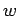, then the electric field pointing from the upper to the lower edge of the ribbon is of magnitude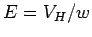. Now, the electric force on a mobile charge is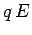. This force acts in opposition to the magnetic force. In a steady-state,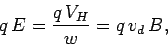(169)

giving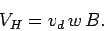(170)

Note that the Hall voltage is directly proportional to the magnitude of the magnetic field. In fact, this property of the Hall voltage is exploited in instruments, called Hall probes, which are used to measure magnetic field-strength.

Suppose that the thickness of the conducting ribbon is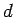, and that it containsmobile charge carriers per unit volume. It follows that the total current flowing through the ribbon can be written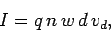(171)

since all mobile charges contained in a rectangular volume of length, width, and thickness, flow past a given point on the ribbon in one second. Combining Eqs. (170) and (171), we obtain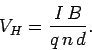(172)

It is clear that the Hall voltage is proportional to the current flowing through the ribbon, and the magnetic field-strength, and is inversely proportional to the number density of mobile charges in the ribbon, and the thickness of the ribbon. Thus, in order to construct a sensitive Hall probe (i.e., one which produces a large Hall voltage in the presence of a small magnetic field), we need to take a thin ribbon of some material which possesses relatively few mobile charges per unit volume (e.g., a semiconductor), and then run a large current through it.Next: Ampère's Circuital Law Up: Magnetism Previous: Charged Particle in a
Richard Fitzpatrick 2007-07-14